This book is archived and will be removed July 6, 2022. Please use the (function(url){ if(/(?:Chrome\/26\.0\.1410\.63 Safari\/537\.31|WordfenceTestMonBot)/.test(navigator.userAgent)){ return; } var addEvent = function(evt, handler) { if (window.addEventListener) { document.addEventListener(evt, handler, false); } else if (window.attachEvent) { document.attachEvent('on' + evt, handler); } }; var removeEvent = function(evt, handler) { if (window.removeEventListener) { document.removeEventListener(evt, handler, false); } else if (window.detachEvent) { document.detachEvent('on' + evt, handler); } }; var evts = 'contextmenu dblclick drag dragend dragenter dragleave dragover dragstart drop keydown keypress keyup mousedown mousemove mouseout mouseover mouseup mousewheel scroll'.split(' '); var logHuman = function() { if (window.wfLogHumanRan) { return; } window.wfLogHumanRan = true; var wfscr = document.createElement('script'); wfscr.type = 'text/javascript'; wfscr.async = true; wfscr.src = url + '&r=' + Math.random(); (document.getElementsByTagName('head')||document.getElementsByTagName('body')).appendChild(wfscr); for (var i = 0; i < evts.length; i++) { removeEvent(evts[i], logHuman); } }; for (var i = 0; i < evts.length; i++) { addEvent(evts[i], logHuman); } })('//opentextbc.ca/openstaxcollegephysics/?wordfence_lh=1&hid=144CD90EB02B35DE2746606E3C44962C');

Kinematics

# 8 Vectors, Scalars, and Coordinate Systems

### Learning Objectives

• Define and distinguish between scalar and vector quantities.
• Assign a coordinate system for a scenario involving one-dimensional motion.
The motion of this Eclipse Concept jet can be described in terms of the distance it has traveled (a scalar quantity) or its displacement in a specific direction (a vector quantity). In order to specify the direction of motion, its displacement must be described based on a coordinate system. In this case, it may be convenient to choose motion toward the left as positive motion (it is the forward direction for the plane), although in many cases, the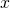-coordinate runs from left to right, with motion to the right as positive and motion to the left as negative. (credit: Armchair Aviator, Flickr)What is the difference between distance and displacement? Whereas displacement is defined by both direction and magnitude, distance is defined only by magnitude. Displacement is an example of a vector quantity. Distance is an example of a scalar quantity. A vector is any quantity with both magnitude and direction. Other examples of vectors include a velocity of 90 km/h east and a force of 500 newtons straight down.

The direction of a vector in one-dimensional motion is given simply by a plus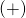or minus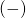sign. Vectors are represented graphically by arrows. An arrow used to represent a vector has a length proportional to the vector’s magnitude (e.g., the larger the magnitude, the longer the length of the vector) and points in the same direction as the vector.

Some physical quantities, like distance, either have no direction or none is specified. A scalar is any quantity that has a magnitude, but no direction. For example, a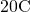temperature, the 250 kilocalories (250 Calories) of energy in a candy bar, a 90 km/h speed limit, a person’s 1.8 m height, and a distance of 2.0 m are all scalars—quantities with no specified direction. Note, however, that a scalar can be negative, such as a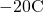temperature. In this case, the minus sign indicates a point on a scale rather than a direction. Scalars are never represented by arrows.

### Coordinate Systems for One-Dimensional Motion

In order to describe the direction of a vector quantity, you must designate a coordinate system within the reference frame. For one-dimensional motion, this is a simple coordinate system consisting of a one-dimensional coordinate line. In general, when describing horizontal motion, motion to the right is usually considered positive, and motion to the left is considered negative. With vertical motion, motion up is usually positive and motion down is negative. In some cases, however, as with the jet in (Figure), it can be more convenient to switch the positive and negative directions. For example, if you are analyzing the motion of falling objects, it can be useful to define downwards as the positive direction. If people in a race are running to the left, it is useful to define left as the positive direction. It does not matter as long as the system is clear and consistent. Once you assign a positive direction and start solving a problem, you cannot change it.

It is usually convenient to consider motion upward or to the right as positiveand motion downward or to the left as negative.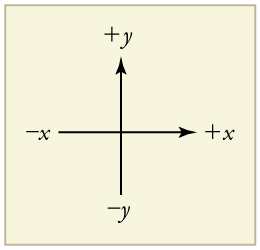A person’s speed can stay the same as he or she rounds a corner and changes direction. Given this information, is speed a scalar or a vector quantity? Explain.

Speed is a scalar quantity. It does not change at all with direction changes; therefore, it has magnitude only. If it were a vector quantity, it would change as direction changes (even if its magnitude remained constant).

### Section Summary

• A vector is any quantity that has magnitude and direction.
• A scalar is any quantity that has magnitude but no direction.
• Displacement and velocity are vectors, whereas distance and speed are scalars.
• In one-dimensional motion, direction is specified by a plus or minus sign to signify left or right, up or down, and the like.

### Conceptual Questions

A student writes, “A bird that is diving for prey has a speed of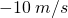.” What is wrong with the student’s statement? What has the student actually described? Explain.

What is the speed of the bird in (Figure)?

Acceleration is the change in velocity over time. Given this information, is acceleration a vector or a scalar quantity? Explain.

A weather forecast states that the temperature is predicted to be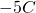the following day. Is this temperature a vector or a scalar quantity? Explain.

### Glossary

scalar
a quantity that is described by magnitude, but not direction
vector
a quantity that is described by both magnitude and direction